# Differential Equations MTH 242 Lecture 08 Dr Manshoor

• Slides: 28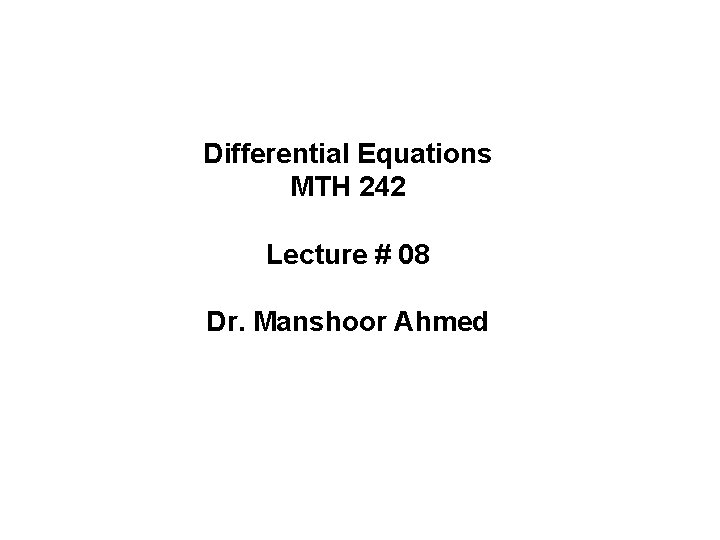Differential Equations MTH 242 Lecture # 08 Dr. Manshoor Ahmed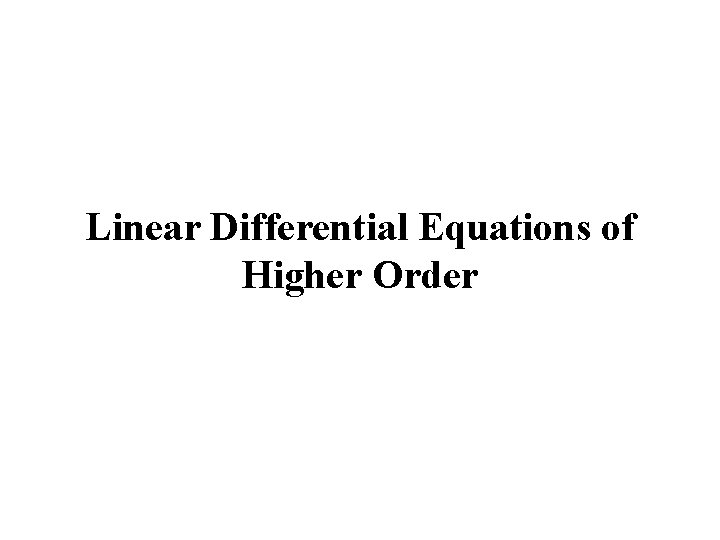Linear Differential Equations of Higher OrderLinear Differential Equations of Higher Order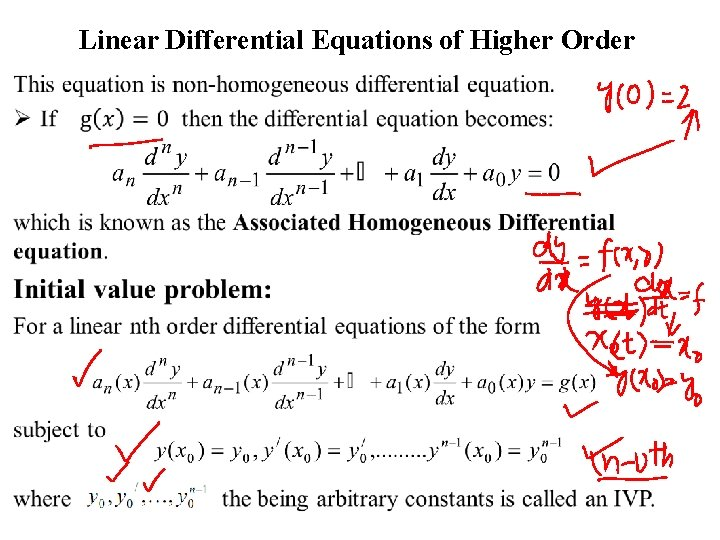Linear Differential Equations of Higher Order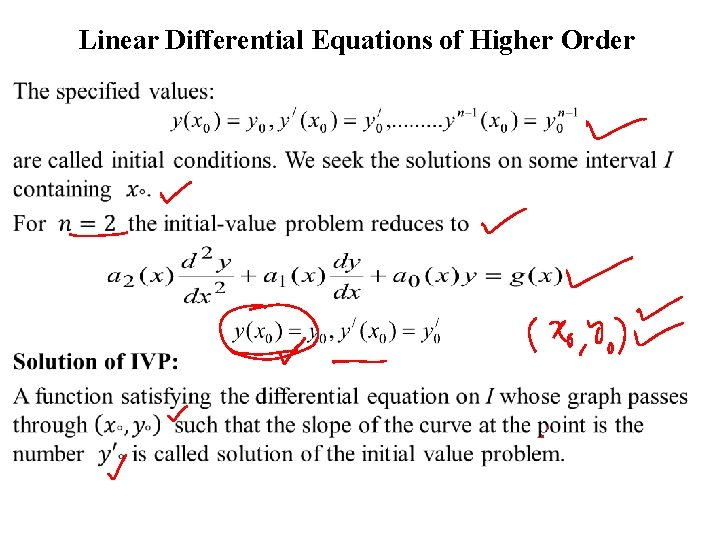Linear Differential Equations of Higher Order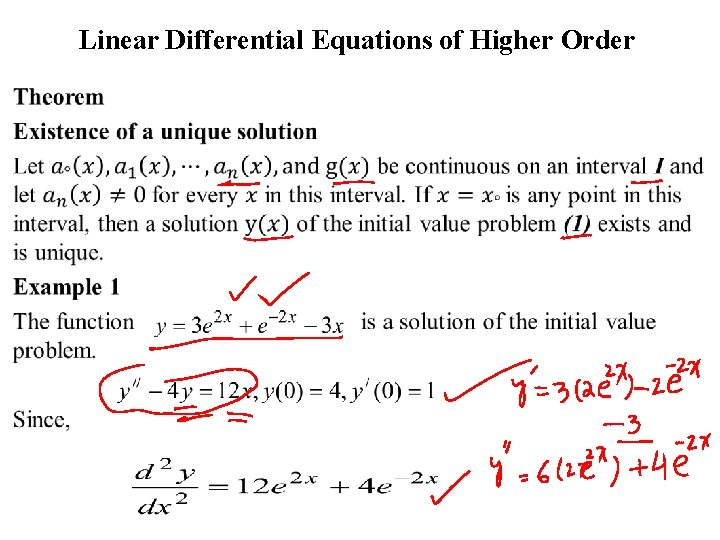Linear Differential Equations of Higher Order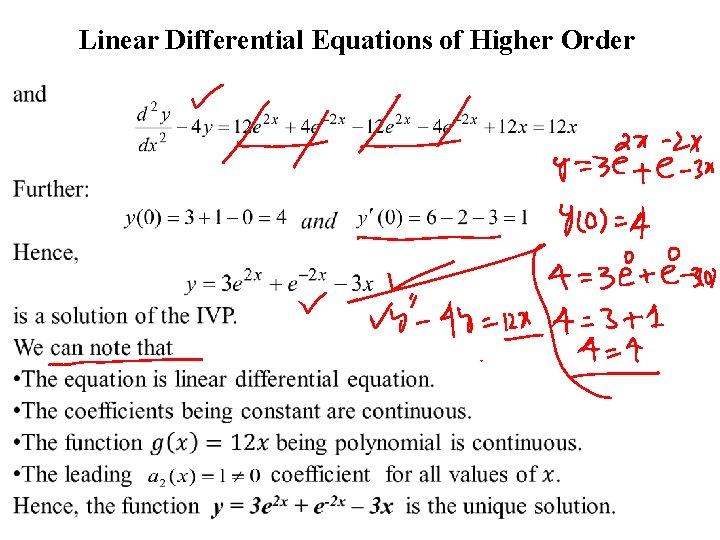Linear Differential Equations of Higher OrderLinear Differential Equations of Higher OrderLinear Differential Equations of Higher OrderLinear Differential Equations of Higher Order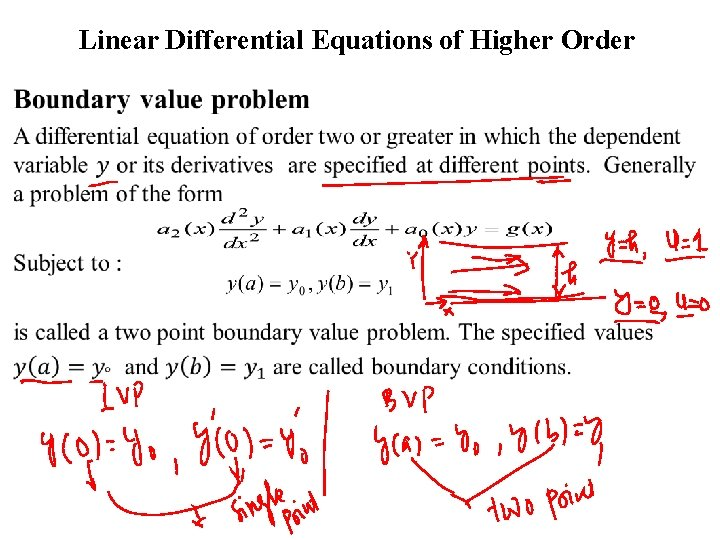Linear Differential Equations of Higher Order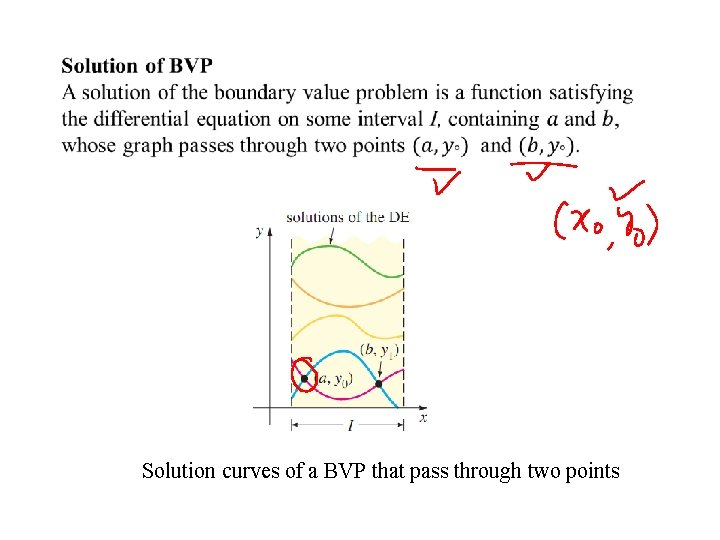Solution curves of a BVP that pass through two pointsLinear Differential Equations of Higher Order Example 1 Consider the function We can prove that this function is a solution of the boundary-value problem Since, Therefore,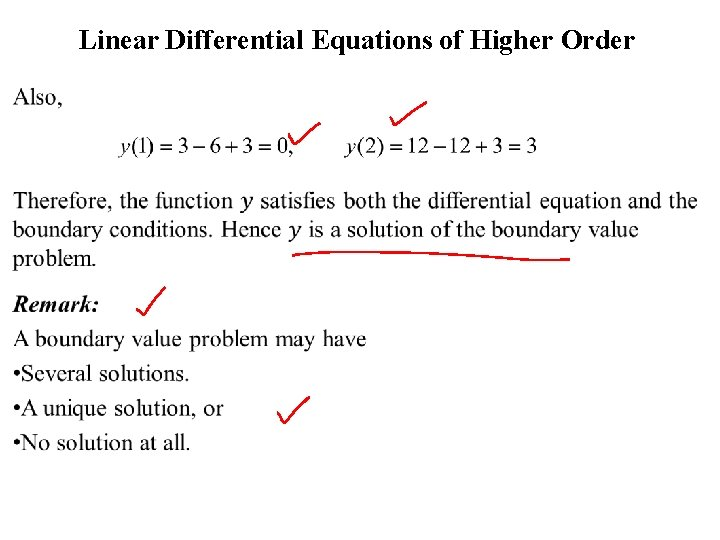Linear Differential Equations of Higher OrderLinear Differential Equations of Higher Order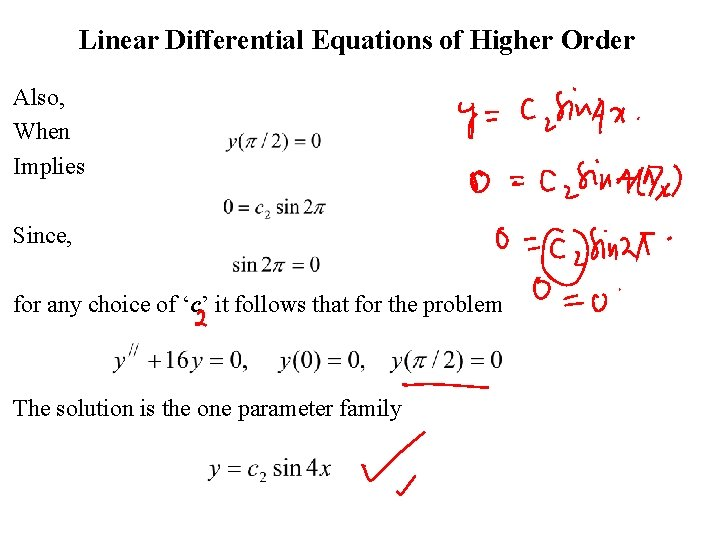Linear Differential Equations of Higher Order Also, When Implies Since, for any choice of ‘c’ it follows that for the problem The solution is the one parameter family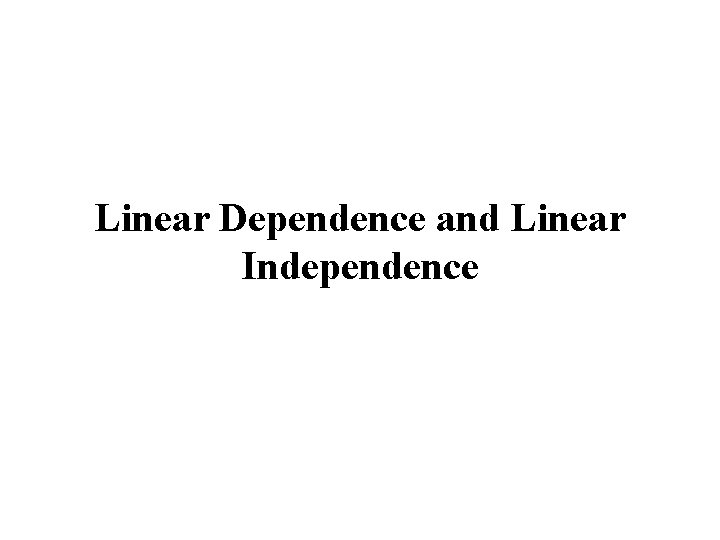Linear Dependence and Linear IndependenceLinear Dependence and Linear IndependenceLinear Dependence and Linear Independence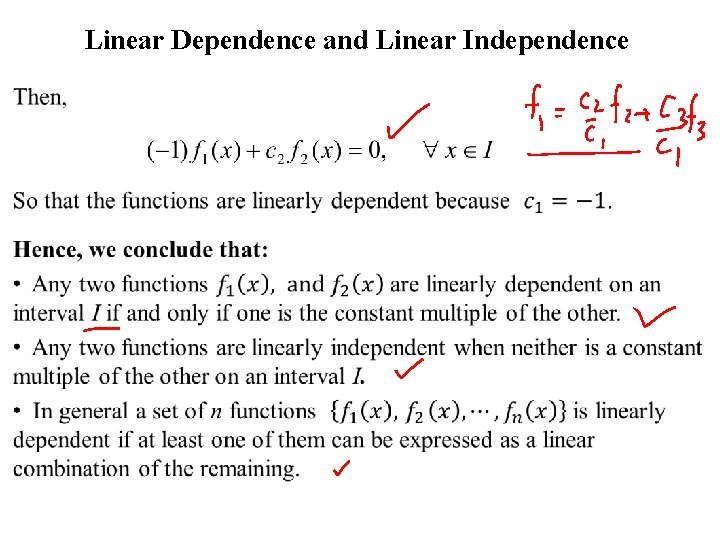Linear Dependence and Linear Independence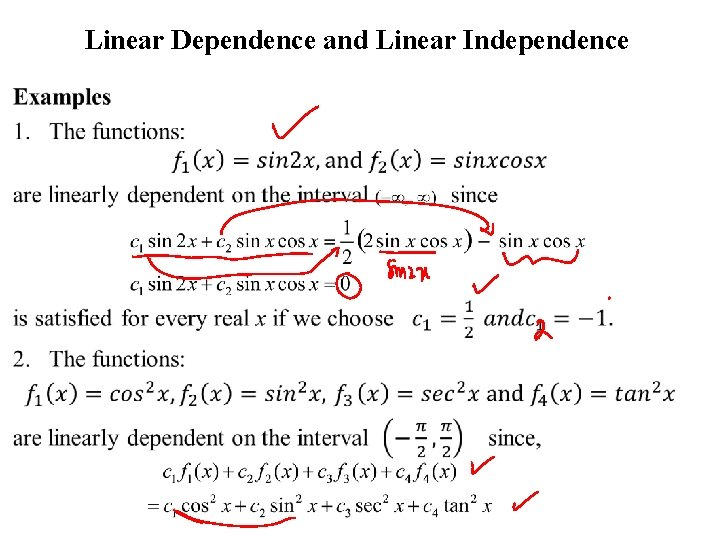Linear Dependence and Linear IndependenceLinear Dependence and Linear IndependenceLinear Dependence and Linear IndependenceLinear Dependence and Linear Independence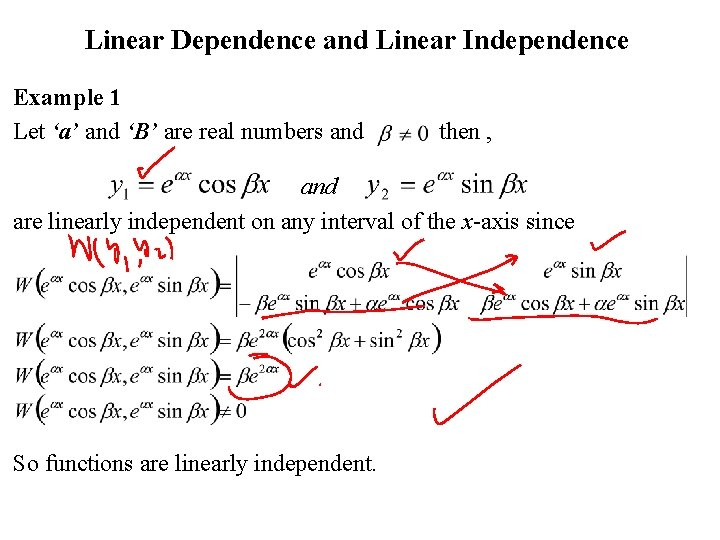Linear Dependence and Linear Independence Example 1 Let ‘a’ and ‘B’ are real numbers and then , and are linearly independent on any interval of the x-axis since So functions are linearly independent.Linear Dependence and Linear IndependenceLinear Dependence and Linear Independence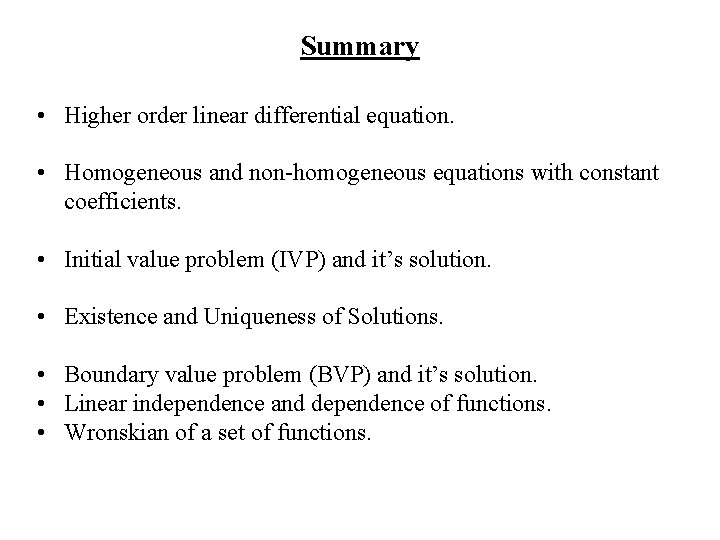Summary • Higher order linear differential equation. • Homogeneous and non-homogeneous equations with constant coefficients. • Initial value problem (IVP) and it’s solution. • Existence and Uniqueness of Solutions. • Boundary value problem (BVP) and it’s solution. • Linear independence and dependence of functions. • Wronskian of a set of functions.